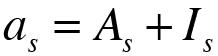LOAN CONSTANT PRINCIPAL REPAYMENTS
 The principal   C0 Number of years   n Number of shorter time units in one year   m Annual payment (1) Semiannual payments (2) Four-monthly payments (3) Quarterly payments (4) Bimonthly payments (6) Monthly payments (12) Weekly payments (52) Daily payments (365) Other: Rate of interest Annual effective rate of interestEffective rate of interest per time unitNominal rate of interest
Calculate more precisely

When you work with loans with constant principal repayments, you have to know the amount of the principal repayments ( As ), dividing the principal ( C0 ) by the number of periods:Once calulated the principal repayments ( As ), you have to calculate the amortization payments ( as ) with the following formula:Now, you have to calculate the interest payments ( Is ) of each period with the following formula:The only thing that need be considered is to always work with the effective rate of interest per time unit ( i(m) ), because of that, if you do not enter this rate of interest, it is necessary to do the following conversion: